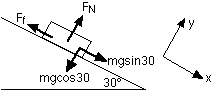## What is the Law Of Conservation Of Mechanical Energy

Question
Spread the love

Last Updated on November 8, 2019 by arkadmin

Mechanical energy is the sum of the potential and kinetic energies in a system. The principle of the conservation of mechanical energy states that the total mechanical energy in a system (i.e., the sum of the potential plus kinetic energies) remains constant as long as the only forces acting are conservative forces. We could use a circular definition and say that a conservative force as a force which doesn’t change the total mechanical energy, which is true, but might shed much light on what it means.

## The conservation of mechanical energy

A good way to think of conservative forces is to consider what happens on a round trip. If the kinetic energy is the same after a round trip, the force is a conservative force, or at least is acting as a conservative force. Consider gravity; you throw a ball straight up, and it leaves your hand with a certain amount of kinetic energy. At the top of its path, it has no kinetic energy, but it has a potential energy equal to the kinetic energy it had when it left your hand. When you catch it again it will have the same kinetic energy as it had when it left your hand. All along the path, the sum of the kinetic and potential energy is a constant, and the kinetic energy at the end, when the ball is back at its starting point, is the same as the kinetic energy at the start, so gravity is a conservative force.

Kinetic friction, on the other hand, is a non-conservative force, because it acts to reduce the mechanical energy in a system. Note that non-conservative forces do not always reduce the mechanical energy; a non-conservative force changes the mechanical energy, so a force that increases the total mechanical energy, like the force provided by a motor or engine, is also a non-conservative force.

### An example

Consider a person on a sled sliding down a 100 m long hill on a 30° incline. The mass is 20 kg, and the person has a velocity of 2 m/s down the hill when they’re at the top. How fast is the person traveling at the bottom of the hill? All we have to worry about is the kinetic energy and the gravitational potential energy; when we add these up at the top and bottom they should be the same, because mechanical energy is being conserved.

At the top: PE = mgh = (20) (9.8) (100sin30°) = 9800 J
KE = 1/2 mv2 = 1/2 (20) (2)2 = 40 J
Total mechanical energy at the top = 9800 + 40 = 9840 J

At the bottom: PE = 0 KE = 1/2 mv2
Total mechanical energy at the bottom = 1/2 mv2

If we conserve mechanical energy, then the mechanical energy at the top must equal what we have at the bottom. This gives:

1/2 mv2 = 9840, so v = 31.3 m/s.

### Modifying the example

Now let’s worry about friction in this problem. Let’s say, because of friction, the velocity at the bottom of the hill is 10 m/s. How much work is done by friction, and what is the coefficient of friction?

The sled has less mechanical energy at the bottom of the slope than at the top because some energy is lost to friction (the energy is transformed into heat, in other words). Now, the energy at the top plus the work done by friction equals the energy at the bottom.

Energy at the top = 9840 J

Energy at the bottom = 1/2 mv2 = 1000 J

Therefore, 9840 + work done by friction = 1000, so friction has done -8840 J worth of work on the sled. The negative sign makes sense because the frictional force is directed opposite to the way the sled is moving.

How large is the frictional force? The work in this case is the negative of the force multiplied by the distance traveled down the slope, which is 100 m. The frictional force must be 88.4 N.

To calculate the coefficient of friction, a free-body diagram is required.In the y-direction, there is no acceleration, so:The coefficient of kinetic friction is the frictional force divided by the normal force, so it’s equal to 88.4 / 169.7 = 0.52.

0
1 year 0 Answers 1085 views 0